# Tutorial – Slope Collision

For Dusk Child 2 I’m going to have slopes in the game, I’ll explain how here 🙂

All slopes are just sprites, placed on the map like any other tile. The key thing is their sprite flags, normally I’d just have a single flag to check to say “is this tile solid or not?” but some parts of a slope tile are solid, and some parts aren’t. So the flags need to describe what kind of slope the tile has, and that looks like this: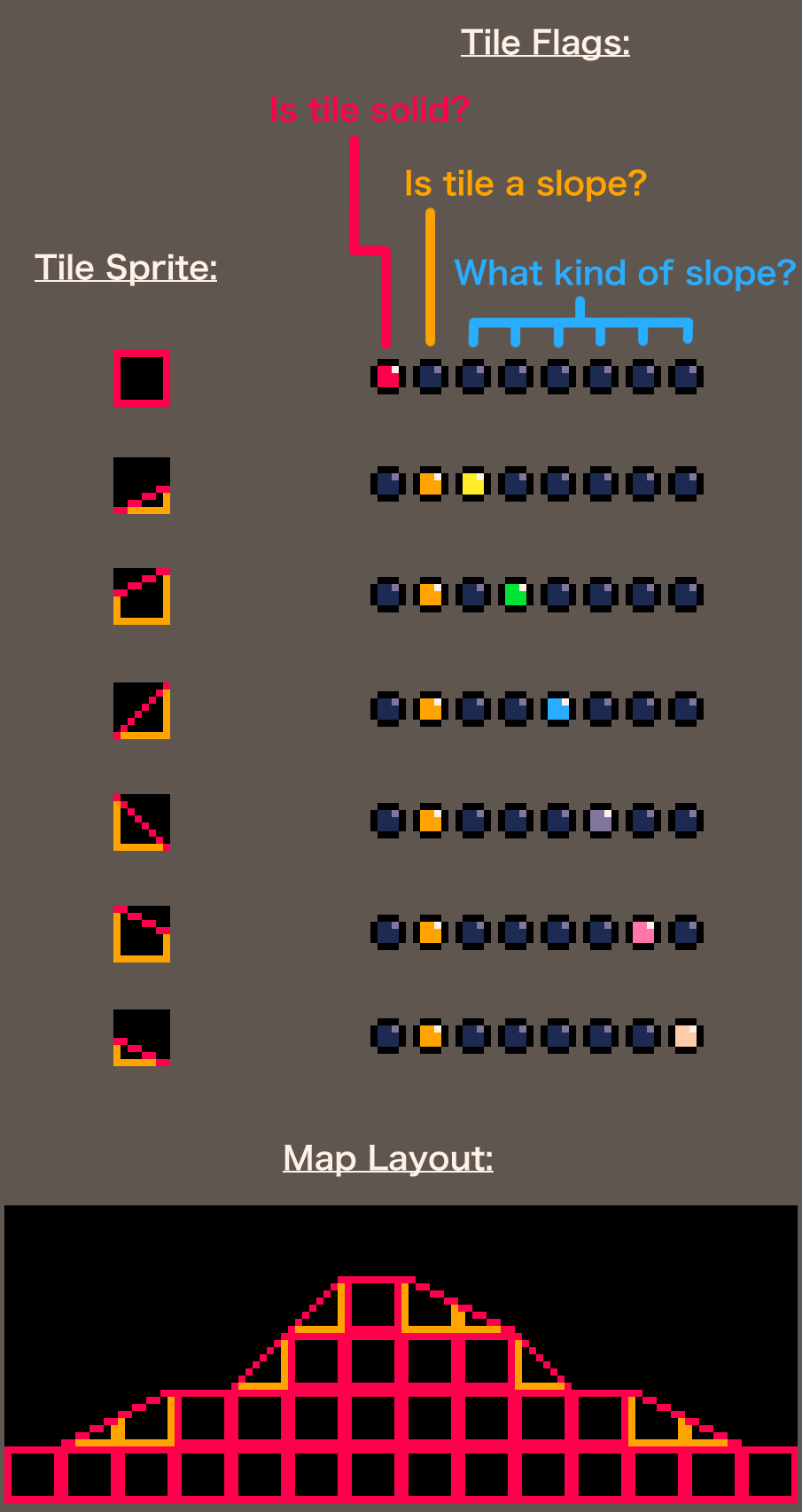The actual sprite appearance don’t really matter too much – it’s the sprite flags that the code will be looking at, so you can go wild with their appearance of course.

Right now Dusk Child 2 looks like this, the surfaces are a little bumpy and uneven to make it look more natural 🙂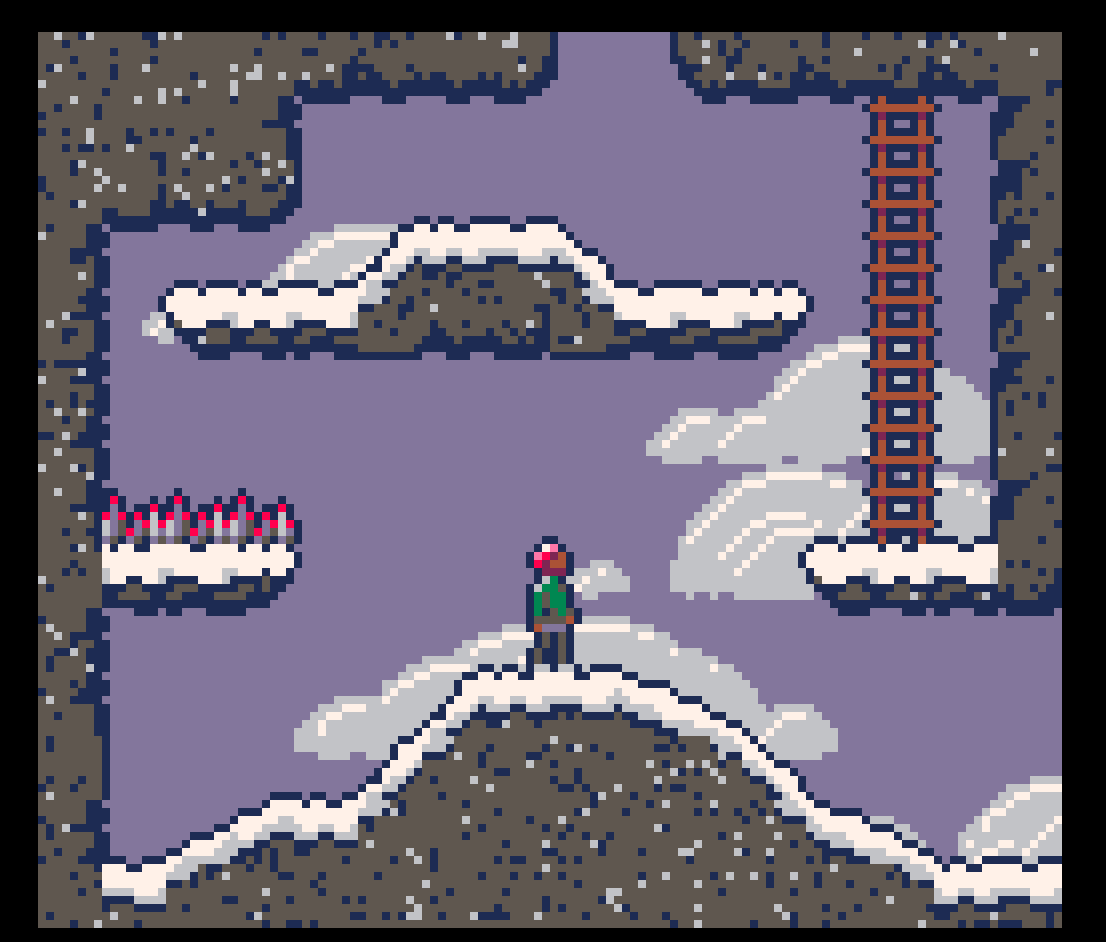It’s all code and math from here, sorry, I’ll try to explain it clearly though!

In order to tell if a point is overlapping a slope or not, first you need to know what kind of tile the point is on. This is a little awkward because it involves converting a game x/y position into map cel_x/cel_y position, I have a function for that though: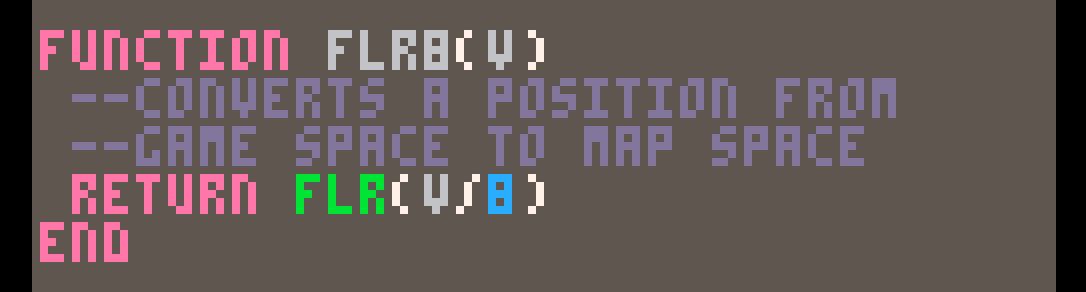It’s not much but with how often I do it, it saves a lot of tokens to make it a function 😉

With that, we can then do MGET( FLR8(X), FLR8(Y) ) to get the sprite ID of any map tile.

Once you have the sprite ID, you can use FGET() to check the sprite’s flags, and know if the tile is a slope and what kind it is.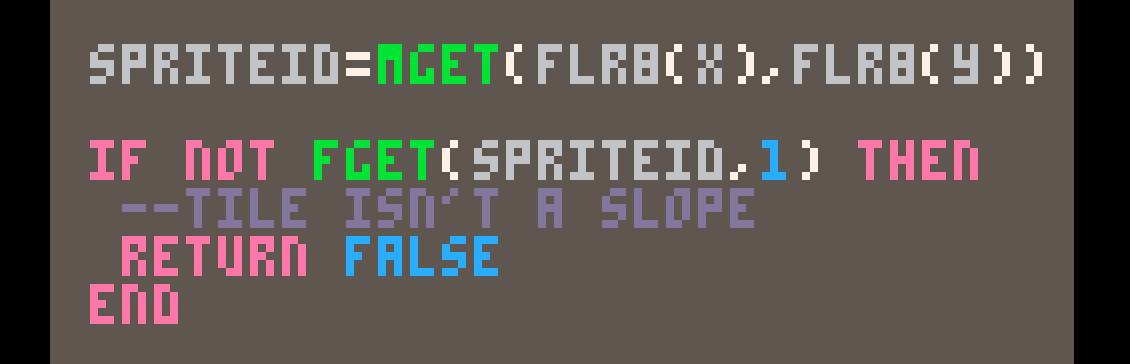So, we can figure out if a point is overlapping a slope tile, how do we figure out if that point is overlapping the slope itself?

We will define our slope by two points; one on the left side of the tile, and one on the right. Our slope is the line between those points – anything above the line is a miss, anything below is a hit.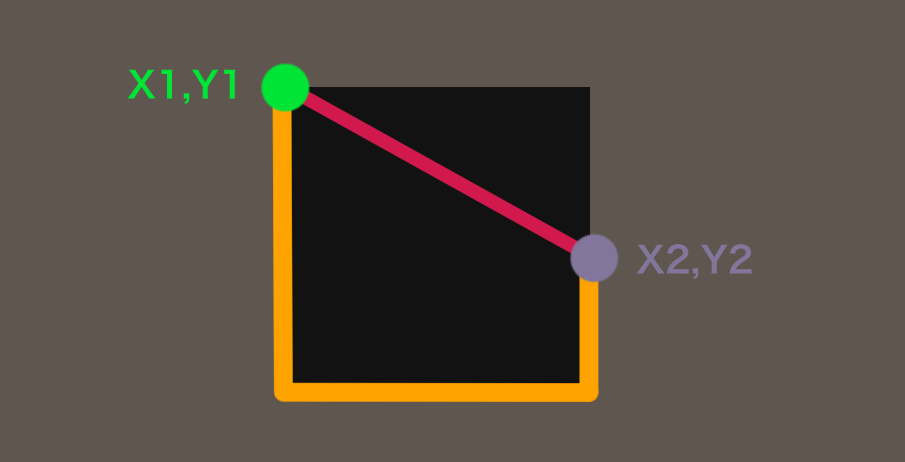We set the values that describe these points (X1, Y1, X2, Y2) based on the tile’s flags – if it’s a steep slope going up (bottom left to top right) that looks like: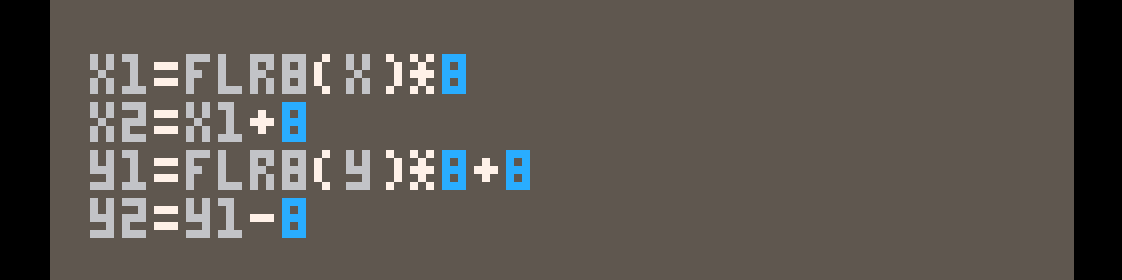In that code, X and Y are the point we are comparing the slope to – so by using FLR8(value)*8 we get the lower bounds of the tile (top or left edge).

You will need to have code to set (X1, Y1, X2, Y2) conditionally for each type of slope.

So just to summarise where we are at, we have:

• X, Y – The point we want to check against the slope
• X1, Y1 – The point on the left side of the slope
• X2, Y2 – The point on the right side of the slope

From here, we just need to find the HEIGHT of the slope at X, if Y is above that then our point isn’t overlapping the slope, otherwise it is.

We know that X is between X1 and X2, but we need to know just how far along, for that I simply do:We divide by 8 so the left side of the tile is 0, the right side is 1. We want T to be in the range of 0-1 because we’ll get the height through linear interpolation (“Lerping”):I’m… not gonna explain interpolation to you, all you really need to know is you plug in A and B, and what you get back will be closer to A if T is near 0, closer to B if T is near 1. Here’s a long confusing wikipedia article if that’s your thing 😛

Anyway, with our distance along the line T we can interpolate between Y1 and Y2 to get the height of the line at X.From here it’s simple, just compare Y to HEIGHT to know if our point overlaps the slope. 🙂

I’ll leave my POINTONSLOPE() function here for you if you want to just copy/paste.

Of course this only lets you check if a point overlaps a slope or not, if you want to do some actual platforming interaction with it… you’re gonna have to work that out yourself for now 😉

<3